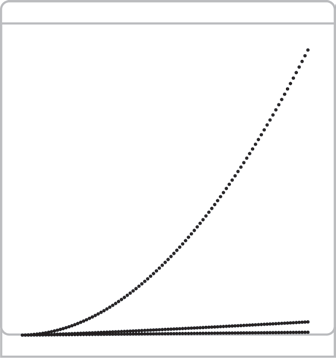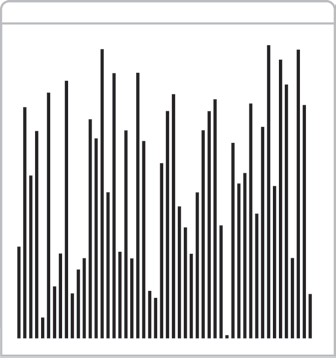# 算法（4th ed）(55)：基础——基础编程模型 3.9.8(输入输出：标准绘图库（控制方法））

 public class StdDraw     static void  setXscale(double x0, double x1) 将 $x$ 的范围设为 $(x_0,x_1)$     static void  setYscale(double y0, double y1) 将 $y$ 的范围设为 $(y_0,y_1)$     static void  setPenRadius(double r) 将画笔的粗细半径设为 $r$     static void  setPenColor(Color c) 将画笔的颜色设为 $c$     static void  setFont(Font f) 将文本字体设为 $f$     static void  setCanvasSize(int w, int h) 将画布窗口的宽和高分别设为 $w$ 和 $h$     static void  clear(Color c) 清空画布并用颜色 $c$ 将其填充     static void  show(int dt) 显示所有图像并暂停 $dt$ 毫秒

int N = 100;StdDraw.setXscale(0, N);StdDraw.setYscale(0, N*N);StdDraw.setPenRadius(.01);for (int i = 1; i <= N; i++){   StdDraw.point(i, i);   StdDraw.point(i, i*i);   StdDraw.point(i, i*Math.log(i));}int N = 50;double[] a = new double[N];for (int i = 0; i < N; i++)   a[i] = StdRandom.random();for (int i = 0; i < N; i++){   double x = 1.0*i/N;   double y = a[i]/2.0;   double rw = 0.5/N;   double rh = a[i]/2.0;   StdDraw.filledRectangle(x, y, rw, rh);}